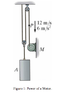# Motor and Pulley System with weight help! (Kind of a Dynamics Problem)

• Engineering
emzee1

## Homework Statement

At the instant shown, point P on the cable has a velocity 12 m/s, which is
increasing at the rate of 6m/s^2. Determine the power input of motor M at this
instant if it operates with an efficiency of 0.8. The mass of the block A is 50kg. I've attached a picture of the problem.

## Homework Equations

F=ma
Power = F*v (F=force, v=velocity)

## The Attempt at a Solution

All I did was make an attempt at drawing free-body diagrams. For the block A of 50kg, I did:

2T-mg=ma; 2T-50(g)=50(6); T= 395 N

Then for point P, I assumed 395 = force of the motor, and thus:
395(12)= 4,740
Input Power = (4,740/.8) = 5,925 W

This is wrong obviously, and was hoping if someone could help me out on where I'm going wrong.

#### Attachments

•Screenshot.png
9.3 KB · Views: 1,098

Homework Helper
You can treat this as conservation of energy if you know how fast the weight is being raised.

You can think your way through this - i.e. does the top pulley matter? What are the speeds of the points on the other two sections of rope at the same height as P?

emzee1
I'm not sure about the last point you made. Is the weight being pulled as fast and at the same rate as point P?

Homework Helper
I'm not sure about the last point you made. Is the weight being pulled as fast and at the same rate as point P?
It's a pulley system - in your experience, is this generally true of pulleys?

You should have done problems involving pulleys by now.
If you don't have experience of pulleys or physics involving pulleys by now: get some!

Off that last point:
See that bit of rope from the ceiling going down to the pulley on the block?
Pick a point on that rope at the same height as point P.
Is that point moving?

emzee1
So, after reviewing my notes. Block A isn't moving as fast/at the same rate as point P. How do I exactly solve for the acceleration for block A? Seeing I have these two equations then:

2T - mg = ma; where I don't know T or a (acceleration) of the block

At point P, the only two forces I can think of is T pointing upwards, and the Force of the motor pointing downwards. But what do I set this equation equal to?

Homework Helper
So, after reviewing my notes. Block A isn't moving as fast/at the same rate as point P. How do I exactly solve for the acceleration for block A?
You'll have an example in your notes for just that.

Put a small mass at point P, which you can set to 0 later on.
So T-F=ma and 2T-Mg=Ma

That may help.

But - considering the pulley system and your notes about pulleys - you should be able to find a short-cut. If you pull on the motor end with speed v, how fast does the block rise?

emzee1
Figured it out, block A has 1/2 the acceleration of point P